## Rainbow Tables: Coverage

A rainbow table is generated by creating (m) chains using randomly picked starting keys. The reduction functions result (or ought to result at least) in evenly distributed new keys. Their is only a probability that a certain key/cipher pair is in the table.

I’ll gradually explain how the coverage can be calculated.

Take m chains, each 1 long to cover a keyspace of N keys. The chance that you can find the key is:When we have 1/2N chains, we’ll subsequently have a P of 1/2, a 50% coverage. Obvious.

When each chain would be 2 in length it gets trickier.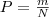still is the chance that we can find the key in the first column (first item of each chain of the table) of the table. The second column isn’t as straight forward. We’ve blindly assumed that each key in the first column is unique, which is true for the first column, but certainly isn’t for the second column. A few chains can merge. The second column is ‘random’.

The chance that a certain key is in a specific chain of the second column is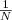. The chance that a certain key is in the second column is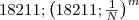(One minus the chance that there isn’t a certain key in a certain chain multiplied by itself the-amount-of-chains times).

The amount of keys covered by this column is the chance that a certain key is in the column times the amount of keys: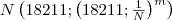.

The chance a certain key is in any of the two columns would be: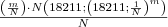The third column’s coverage can be calculated by taking the unique keys in the second column as m was taken for the amount of unique keys for the first column. With each chain t in length this formula applies: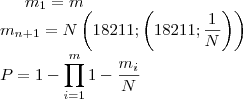## Rainbow Tables: Introduction

A rainbow table is a data structure with which it is possible to retreive the key used to generate a ciphertext with a fixed plaintext. One common application is to reverse (password) hashes; which can be seen as cipher functions who take the message as the key and the ciphertext as the generated hash.

One important thing to note is that all keys and ciphertext pairs stored in the table have been precomputed during generation of the table. Generating a table that stores 1 miljon md5 password hashes, requires all those passwords to be hashed during precompution.

I’ve been doing some research about rainbow tables and I think it will be usefull to share some of my experiences.

But before I can dive into the matter I first got to explain how rainbow tables work. (or you can read the ‘official’ paper about rainbow tables by Philippe Oechslin).

Precomputing a significant amount of key/ciphertext (password/password hash) pairs is fairly easy. The issue is that a significant amount is fairly large, 237 for alphanumerical windows password hashes to give a real-life example. Because it isn’t practical to store 237 pairs of key/ciphertext’s (~2TB) on your computer; rainbow tables store chains of key/ciphertext’s pairs, more precisely they store only the starting and ending key of a chain.

Each chain starts with a key (randomly generated; in our example a password). The next item in the chain is the ciphertext which is calculated by the cipher (in our example the hash for the password). The next item is a key (password) again, which was generated from the ciphertext (hash) by a reduction function. This reduction function can be almost everything, it can be a XOR hash, it can be CRC32, as long as it changes the (larger) cipher text into a new key.

k1 — cipher –> C1 — reduction function 1–> k2 — cipher –> C2 — reduction function 2 –>…

Each chain has got a fixed length (t). In the table only the first and the last key are stored (k1 and kt).

To look up a certain ciphertext, the reduction function is applied on the cipher text and all the end-keys of all chains are checked whether they match. If one of them matches you can regenerate the chain from the begin key stored allong the end key of the chain, and you’ll find the key that when applied the reduction and the cipher on will result in your ciphertext (which is the last ciphertext in the chain). When no end key matches, the cipher and reduction functions are applied to generate a new key, and all the end key’s are checked again. If one of the end key’s matches you know that your ciphertext is in the chain in the second-last position. Repeat this t times for each position in the chain.

It sounds straight forward, but there are some issues that appear:

• Loops, there are some cases where the reduction function generates a key which when being applied the cipher function will become the first plain text. (K = R(S(K)), where R is the reduction function, and S is the cipher). This will cause a the rest of the chain to be useless.
• Merging chains/False alarms, because the ciphertext is larger than the key there will likely be a lot of chains with the same end point, this reduces the total coverage of the table and will cause a lot of additional time spent on the ‘false alarms’ caused by the fact that you have to check each matching endpoint, even if the starting key is the wrong branch.

To avoid loops; avoid merging chains a bit more and to increase lookup speed, rainbow tables use rainbow chains: each column in the table uses a different reduction function.

Because we use a different reduction function for each ciphertext in the chain there can’t be loops, or at least not loops that cause redundant data, because a certain ciphertext will become a different key in a different column of the chain.

The amount of merging chains is also cut by t times, because a certain key in a chain will only lead to the same chain when it is in the same place of the chain.

This is a very superficial explanation, read more (when you’re interested) in the original paper.

## The problem with rainbow tables

Rainbow tables (Making a Faster Cryptanalytic Time-Memory Trade-Off) work fairly well, although – because they use different regression functions in the chains – they contain a lot of redundant data.

The example table in the paper (lanmanager hash; 8 char. alpha; 4666×38223872) contains a lot of redundant data, which can be calculated:

>>> CalcRedundancy(4666, 38223872, CalcN(26, 1, 7)) 21.50866258033486

The 300mb table would be stored in a 20mb table if the regression function was to be perfect.

The problem is that to make a regression function perfect, it should be ‘in paralel’ with the cipher function, which isn’t an option for most of these are engineered to prevent this.

Adding variable length chains back into the rainbow chains could allow exploiting existing chains more efficiently.

This probably sounds messy and to be honoust this idea is still a bit messy.

I`ll write something decent along the way.